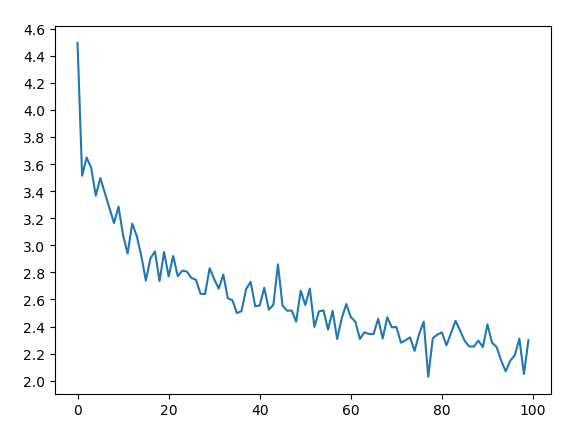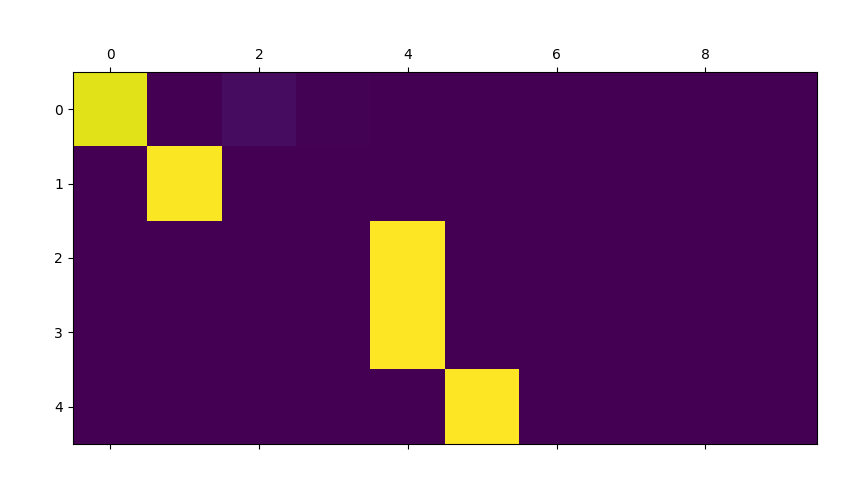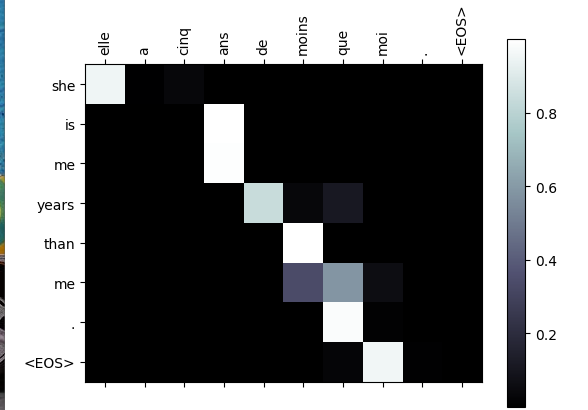# 简介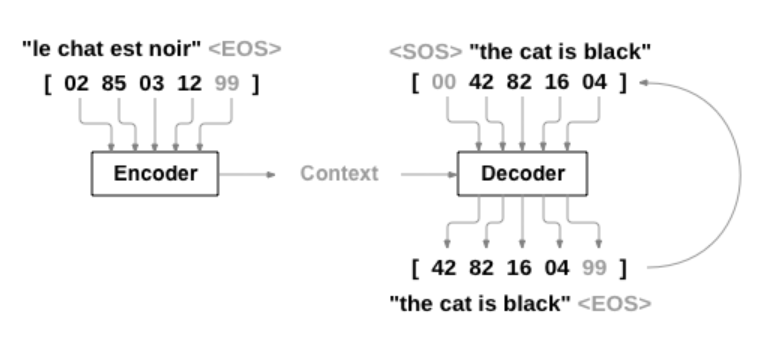# 数据集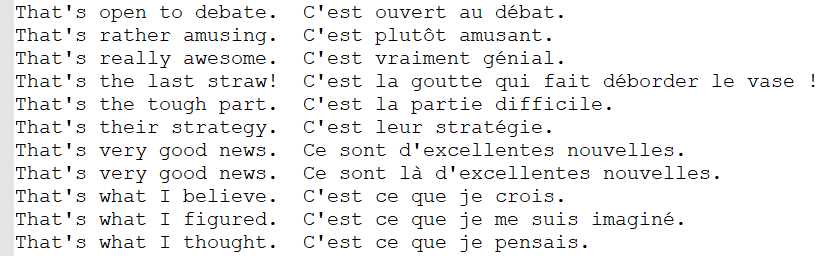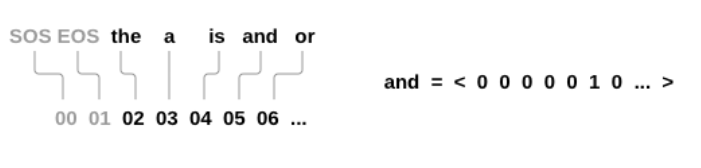SOS_token = 0
EOS_token = 1

class Lang:
def __init__(self, name):
self.name = name
self.word2index = {}
self.word2count = {}
self.index2word = {0: "SOS", 1: "EOS"}
self.n_words = 2  # Count SOS and EOS

for word in sentence.split(' '):

if word not in self.word2index:
self.word2index[word] = self.n_words
self.word2count[word] = 1
self.index2word[self.n_words] = word
self.n_words += 1
else:
self.word2count[word] += 1

def readLangs(lang1, lang2, reverse=False):

# Read the file and split into lines
lines = open('data/%s-%s.txt' % (lang1, lang2), encoding='utf-8').\

# Split every line into pairs and normalize
pairs = [[normalizeString(s) for s in l.split('\t')] for l in lines]

# Reverse pairs, make Lang instances
if reverse:
pairs = [list(reversed(p)) for p in pairs]
input_lang = Lang(lang2)
output_lang = Lang(lang1)
else:
input_lang = Lang(lang1)
output_lang = Lang(lang2)

return input_lang, output_lang, pairs

1.读取文本文件并拆分成行，将行拆分成对
2.使文本标准化，按照长度和内容进行过滤
3.从成对的句子中构建单词列表

# Seq2Seq模型

Seq2Seq(Sequence to Sequence network or Encoder Decoder network)是由两个称为编码器和解码器的RNN组成的模型。 编码器读取输入序列并输出单个矢量，解码器读取该矢量以产生输出序列。

## The Encoder

seq2seq网络的编码器是一个RNN，它为输入句子中的每个单词输出一些值。 对于每个输入单词，编码器输出一个向量和一个隐藏状态，这个隐藏状态和下一个单词构成下一步的输入。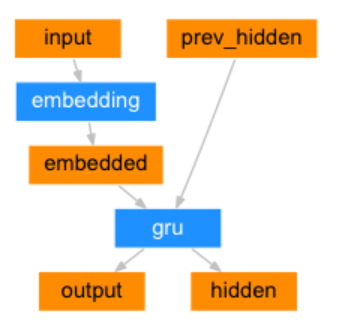class EncoderRNN(nn.Module):
def  __init__(self, input_size, hidden_size):
super(EncoderRNN, self).__init__()
self.hidden_size = hidden_size

self.embedding = nn.Embedding(input_size, hidden_size)
self.gru = nn.GRU(hidden_size, hidden_size)

def forward(self, input, hidden):
embedded = self.embedding(input).view(1, 1, -1)
output = embedded
output, hidden = self.gru(output, hidden)
return output, hidden

def initHidden(self):
result = Variable(torch.zeros(1, 1, self.hidden_size))
return result


## The Decoder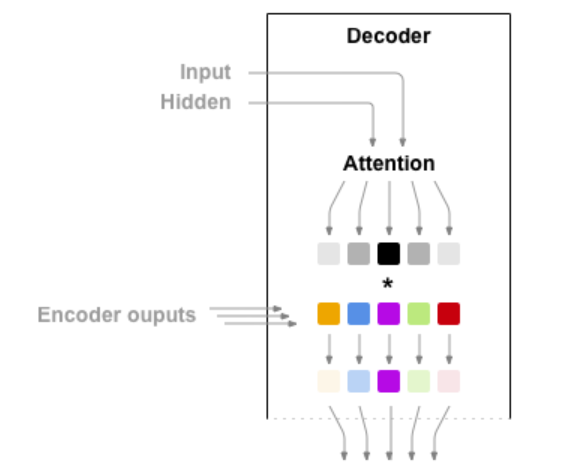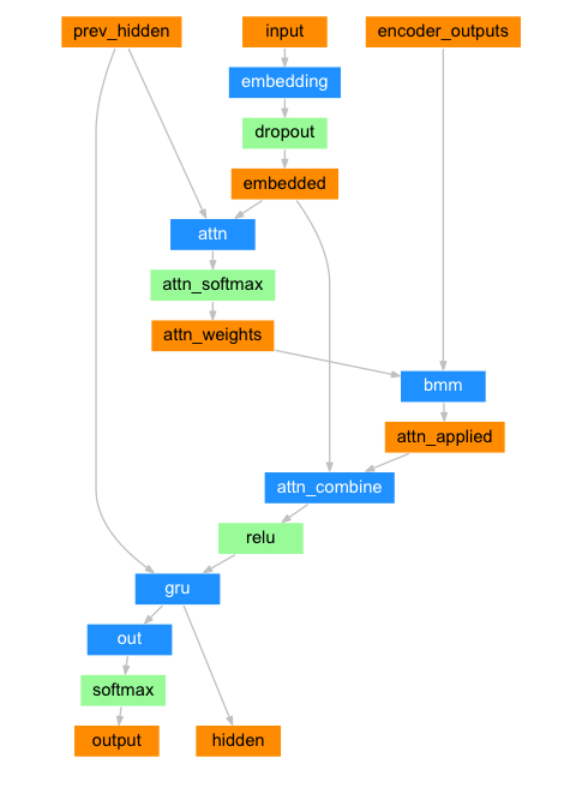class AttnDecoderRNN(nn.Module):
def __init__(self, hidden_size, output_size, dropout_p=0.1, max_length=MAX_LENGTH):
super(AttnDecoderRNN, self).__init__()
self.hidden_size = hidden_size
self.output_size = output_size
self.dropout_p = dropout_p
self.max_length = max_length

self.embedding = nn.Embedding(self.output_size, self.hidden_size)
self.attn = nn.Linear(self.hidden_size * 2, self.max_length)
self.attn_combine = nn.Linear(self.hidden_size * 2, self.hidden_size)
self.dropout = nn.Dropout(self.dropout_p)
self.gru = nn.GRU(self.hidden_size, self.hidden_size)
self.out = nn.Linear(self.hidden_size, self.output_size)

def forward(self, input, hidden, encoder_outputs):
embedded = self.embedding(input).view(1, 1, -1)
embedded = self.dropout(embedded)
attn_weights = F.softmax(
self.attn(torch.cat((embedded, hidden), 1)), dim=1)
attn_applied = torch.bmm(attn_weights.unsqueeze(0),
encoder_outputs.unsqueeze(0))
output = torch.cat((embedded, attn_applied), 1)
output = self.attn_combine(output).unsqueeze(0)
output = F.relu(output)
output, hidden = self.gru(output, hidden)
output = F.log_softmax(self.out(output), dim=1)
return output, hidden, attn_weights

def initHidden(self):
result = Variable(torch.zeros(1, 1, self.hidden_size))
return result

## 训练和测试模型

loss 图: